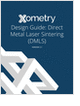MaterialsDesign CenterProcessesUnit ConversionFormulasMathematicsCalculatorsDiscussion ForumTrade PublicationsDirectory Service
Metal 3D Printing Design Guide

Direct Metal Laser Sintering (DMLS) 3D printing for parts with reduced cost and little waste.

Learn the best principles to negotiate the salary you deserve!

3D Scanners

A white paper to assist in the evaluation of 3D scanning hardware solutions.

Injection Molding Design Guide

Guide for high quality and cost-effective plastic injection molding.

more free publicationsGlossary » Units » Force » A.u. of ForceA.u. of Force (a.u.) is a unit in the category of Force. It is also known as atomic units, u.a., ua. This unit is commonly used in the a.u. unit system. A.u. of Force (a.u.) has a dimension of MLT-2 where M is mass, L is length, and T is time. It can be converted to the corresponding standard SI unit N by multiplying its value by a factor of 8.238729477E-008.
Note that the seven base dimensions are M (Mass), L (Length), T (Time), Q (Temperature), N (Aamount of Substance), I (Electric Current), and J (Luminous Intensity).

Other units in the category of Force include Crinal (crinal), Dyne (dyn), Gram Force (gf), Joule Per Centimeter (J/cm), Kilogram Force (kgf, kgp), Kilogram Meter Per Square Second (kg-m/s2), Kilopound Force (kip Force) (kipf), Newton (N), Ounce Force (av.) (ozf (av.)), Pound Force (lbf (av.), lbf), Pound Force Foot Per Inch (lbf-ft/in), Poundal (pdl), Slug Foot Per Square Second (slug-ft/s2), Slug Force, Square Foot Inch of Mercury (0°C) (ft2-inHg (0 °C)), Square Foot Inch of Mercury (15.56°C) (ft2-inHg (15.56 °C)), Ton Force (long), Ton Force (metric), and Ton Force (short).N/ARelated PagesMetal 3D Printing Design Guide

Direct Metal Laser Sintering (DMLS) 3D printing for parts with reduced cost and little waste.